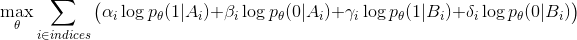# Optimization Problem Implementation

Hi,

I am trying to implement a method from a paper, which requires to solve eqation. The p_\theta is a CNN, which has a sigmoid function at the end, \alpha, \beta, … are known values, \theta are parameters of the CNN, A_i and B_i are images for i-th sample. It is suggested to use weighted BCE Loss to solve this, but I am struggling to work out how to do this in PyTorch. Two of my ideas are (iterate over samples and):

1. Use negative of the inner (max and sum) function as a (custom) loss to backpropagate
2. Use \alpha as a weight for BCELoss function, where the target tensor is one, \beta as a weight for BCELoss, where the target tensor is zero etc.

But… both of these methods should lead to the same solution, is this not a true? Is this solution correct, or am I missing something?

2. I am not sure you can set the weights this way with the BCELoss we have. But the formula above is a bit unclear on what are different samples in a batch and what is not. But the function we have might enforce that beta = 1 - alpha and delta = 1-gamma.